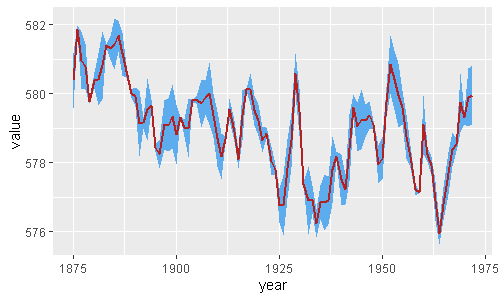Want to share your content on R-bloggers? click here if you have a blog, or here if you don't.Yesterday I was asked to easily plot confidence intervals at ggplot2 chart. Then I came up with this shadowing ggplot2 feature called `geom_ribbon()`.

It’s not a trivial issue as long as you need to gather your data in order to achieve a tidy format. When you already have this data frame, all you need is geom_ribbon().

By using the following commented code you are able to show not only your point estimated forecast but also its confidence or prediction intervals.

```library(tidyverse)
huron <- data.frame(year = 1875:1972,
value = LakeHuron,
std = runif(length(LakeHuron),0,1))

huron %>%
ggplot(aes(year, value)) +
geom_ribbon(aes(ymin = value - std,
ymax = value + std), # shadowing cnf intervals
fill = "steelblue2") +
geom_line(color = "firebrick",
size = 1) # point estimate```

For a multi-line plot, you should include the colour and group aesthetic as follows:

```library(tidyverse)
huron <- data.frame(year = rep(1875:1972,2),
group = c(rep("a",98),rep("b",98)),
value = c(LakeHuron, LakeHuron + 5),
std = runif(length(LakeHuron)*2,0,1))

huron %>%
ggplot(aes(year, value, fill = group)) +
geom_ribbon(aes(ymin = value - std,
ymax = value + std,
group=group),
fill = "steelblue2") +
geom_line(color = "firebrick", size = 1)```You can see related R visualizations and tips on TypeThePipe